## research papersBIOLOGICALCRYSTALLOGRAPHY
ISSN: 1399-0047
Volume 56| Part 10| October 2000| Pages 1223-1232

## Low-resolution ab initio phasing: problems and advances

aInstitute of Mathematical Problems of Biology, Russian Academy of Sciences, Pushchino, Moscow Region 142292, Russia,bLCM3B, UPRESA 7036 CNRS, Faculté des Sciences, Université Henry Poincaré Nancy I, 54506 Vandoeuvre-lès-Nancy, France, and cUPR de Biologie Structurale, IGBMC, BP 163, 67404 Illkirch CEDEX, CU de Strasbourg, France
*Correspondence e-mail: sacha@lcm3b.u-nancy.fr

(Received 31 January 2000; accepted 19 July 2000)

If only native amplitudes are used for structure determination, then additional theoretical' information is necessary to determine their phases. For use in a phasing procedure, this information can be formulated as a selection criterion (figure of merit) which assigns a reliability weight to every trial phase set and distinguishes the closest ones to the true phase set. Different types of additional information may be tested as a selection criterion: electron-density histograms, connectivity properties, statistical likelihood, atomicity etc. A common feature of such criteria is that they do not unambiguously judge the phase quality at low resolution. Nevertheless, the selection of the phase sets with best criterion values increases the ratio of good phase sets in the ensemble considered. An approximate solution of the phase problem may then be found by averaging the selected phase sets. Cluster analysis of the selected phase sets and averaging within clusters allow further improvement of this solution.

### 1. Introduction

The development of classical direct methods for successful ab initio phasing of small proteins (Miller & Weeks, 1998; Sheldrick, 1998; Woolfson, 1998) has not reached yet the realm of large macromolecules, where the necessary very high resolution diffraction data are not usually available. Therefore, the elaboration of alternative methods of ab initio phasing which could be applied in this case is necessary. The methods of phasing a relatively small number of very low resolution (VLR) reflections occupy a special place among phasing approaches. On one hand, the information which can be extracted from VLR Fourier syntheses is essentially limited and mostly concerns the macromolecular envelope and its position in the unit cell. On the other hand, these VLR phases may be used as a starting point for phase-extension procedures. The knowledge of the object shape and position can facilitate the use of alternative sources of information (e.g. electron-microscopy reconstructed images) together with X-­ray diffraction data.

Obviously, the lack of experimental information when working only with low-resolution data must be compensated by additional hypotheses concerning the nature of the object studied; the choice of a proper hypothesis plays a key role in the success of the phasing procedure. In this paper, we discuss the results of studies undertaken over the last decade regarding the usefulness of different kinds of such hypotheses and reveal their common features. At first glance, these features are disappointing; none of the existing criteria allows unambiguous judgement of the phase-set quality. Nevertheless, the criteria considered possess some phasing power and a method is suggested whereby this phasing power could be used for ab initio phasing.

### 2. Definitions

Some general definitions are given below to simplify the discussion. The aim of this paper is to discuss possible approaches to the solution of the phase problem, i.e. to determine the values {φ(h)}hS of structure-factor phases for some set S of reflections. We suppose below that S is chosen and fixed, that it contains all low-resolution reflections up to a resolution d and that all corresponding structure-factor magnitudes {Fobs(h)}hS are known. To find the best solution, a large variety of different trial phase sets are usually studied. We call a variant (of the solution of the phase problem) any set of values {φ(h)}hS that matches the symmetry restrictions on the phase values for centric reflections. Any given set of variants is called a population (of variants). The general strategy will be to consider first a large population (e.g. a randomly generated one) and to select from it the variants which are most likely to be of reasonable quality. Obviously, the selection criterion plays a crucial role in this process and the main objective is to discuss possible criteria and their success rate. In classical direct methods the selection criterion is traditionally called the figure of merit', but we reserve here the term figure of merit for the weights that reflect the reliability of individual phases (Blow & Crick, 1959) and define the selection criterion value as a number which is linked to the phase set as the whole.

The variants may be formally considered as points in a multidimensional configurational space and different metrics may be used to measure the closeness of two variants. We start below from the phase correlation, which is defined as the map correlation coefficient (Lunin & Woolfson, 1993) corresponding to two Fourier syntheses ρ1(r) and ρ2(r) calculated from the same set of observed magnitudes (without the F000 term), but with different phases,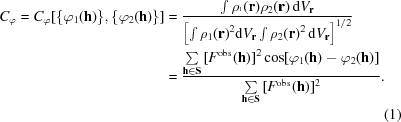Two phase sets, while appearing to be different, may result in Fourier syntheses which differ only by a permitted origin shift (and/or enantiomorph choice). Such variants must be considered as equivalent and the best map alignment with respect to the choice of the origin and enantiomorph must be obtained before calculating the value Cφ (Lunin et al., 1990; Lunin & Lunina, 1996).

Sometimes it is more convenient to discuss the dissimilarity of variants rather than their correlation. We define the distance between variants as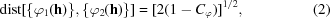so that the minimal possible distance is zero (for Cφ = 1) and the maximal distance is 2 (for Cφ = −1). Neither the phase correlation nor distance depend on the scale of the observed magnitudes.

To check the utility of a phasing procedure, tests are usually performed using observed magnitudes corresponding to known structures. In such cases, the phases {φmodel(h)}hS calculated from the refined atomic model may be considered to be the true solution and used to judge the phasing success. When analysing a population of variants, we call those which have a high phase correlation (with respect to the true phases) good variants and those with a relatively low correlation are called bad variants.

This paper is devoted to the problem of phasing of a few dozens of reflections of the central zone in reciprocal space. A formal resolution corresponding to such set of reflections is different for different test objects, but in all cases considered it does not exceed the 15 Å limit, where the influence of the solvent content becomes highly irregular (Podjarny & Urzhumtsev, 1997).

We consider an interpretable Fourier synthesis as the main goal of phasing. Thus, we consider the map correlation coefficient (1) as the most adequate indicator of success. A more traditional mean phase error may be misleading at low resolution, because usually some very strong reflections are present in this zone. Small phase errors for such reflections may influence the map quality much more then relatively large phase errors in weak reflections. Therefore, a weighted phase difference (1) seems to be a more reliable figure when a small number of reflections are involved in work.

### 3. Ab initio phasing procedure

We start with general notes about the phasing approach. More details are discussed later, together with test structure examples.

At the beginning of a structure determination there is no preference for any phase set, so different variants must be considered as equally possible candidates for the solution of the phase problem. In the following we start from a randomly generated population of variants (Lunin et al., 1990; Woolfson & Yao, 1990); note that more sophisticated approaches to the choice of a starting population may be applied (Lunin et al., 1995; Gilmore et al., 1999). If the random starting population is large enough, there exists a possibility that the population contains several variants that are good, i.e. close to the true solution, and the problem is to distinguish them from the rest. However, this possibility falls exponentially with the number of reflections included in S, so only a relatively small number of reflections may be considered at the first stage. If approximate values are known for some phases, then preference may be given to variants possessing values close to these known values. This can be performed by fixing the corresponding phases, by using a non-uniform probability distribution when generating phases randomly or by using a more sophisticated procedure to generate the phase values (Lunin et al., 1998). In such a case, the number of reflections considered may be increased gradually in a phase-extension procedure.

In order to select good variants from a given population, it is necessary to have some selection criterion for recognizing these variants. Such criteria might be based on general properties of the true phase set which can be established before any atomic coordinates are found. The choice of such a criterion is the crucial step in ab initio phasing and several possibilities are discussed below. For any particular selection criterion, the natural idea is to choose the variant with the best criterion value. Unfortunately, this idea fails! This is demonstrated in tests below with a variety of different selection criteria; none allows the correct phase set to be determined unambiguously. The usual case is that the best variant in the population does not have the best value of the selection criterion. On the contrary, the best selection-criterion value may correspond to a totally wrong phase set. Nevertheless, the criteria studied are not useless. In many cases there exists a statistical tendency for good variants to have better criterion values than bad ones. To exploit this tendency, we formulate our task not as one of finding the variant with the best criterion value, but rather as one of selecting all variants with reasonable criterion values. It must be noted that the best variants may be lost in this process and some wrong variants may be retained. Nevertheless, this procedure increases the concentration of good variants in the selected population in comparison with the initial population.

This enrichment procedure tends to concentrate the variants in the vicinity of the true solution, so that the point in configurational space with the largest concentration of selected variants may serve as a starting approximation for the solution of the phase problem. A more accurate approximation is achieved by averaging the selected variants to obtain the centroid phases and individual figures of merit for these phases,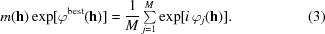Here, φj(h) is the phase of the h-indexed reflection in the jth selected variant. As mentioned above, the optimal alignment of selected variants must be performed before averaging.

This simple averaging procedure often results in a reasonable solution of the phase problem. Nevertheless, it was sometimes found that several centres of concentration appear. Cluster-analysis methods allow the distribution of the selected variants in configurational space to be studied more precisely (Lunin et al., 1990, 1995). These methods show how the points are distributed in a multidimensional space. They are either distributed almost uniformly, form a compact group (a cluster) or fall into several compact clusters etc. The input information for cluster analysis is the matrix of variant-to-variant distances calculated in (2), so this analysis does not require knowledge of the true solution. If several clusters are revealed in the cluster analysis, then averaging the variants in every cluster separately via (3) provides several alternative solutions of the phase problem. These alternatives may be used for the construction of a phasing tree (Bricogne & Gilmore, 1990) or resolved by the use of cluster tests (Lunin et al., 1998).

Fig. 1summarizes the suggested procedure for ab initio phasing. In the following sections, examples of the suggested approach are shown for several test structures. It is worth noting that all these tests were performed with experimental data sets and that these data sets contained all the very low resolution reflections.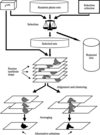Figure 1 Flow chart of the phasing procedure.

### 4. Histogram-based phasing

#### 4.1. Fourier syntheses histograms

The first example of a selection criterion is the histogram-based one (Lunin et al., 1990). The histogram of a Fourier synthesis indicates which values are present and how frequently these values appear in the synthesis. Let a function ρ(r) be calculated at N grid points in the unit cell. Assume the interval (ρmin, ρmax) of possible ρ(r) values is divided into K equal parts (bins) and for every bin the frequency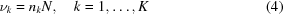is calculated, where nk is the number of grid points with ρ(r) values belonging to the kth bin. We shall call the set of frequencies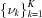the histogram corresponding to the function ρ(r) and call the standard histogram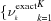the histogram corresponding to the Fourier synthesis calculated with the observed magnitudes and the true phases. The standard histogram depends on the resolution of the synthesis and is sensitive to phase errors. The standard histogram at a particular resolution can be predicted before phases are determined. Several approaches for the use of histogram information were suggested independently (Lunin, 1988, 1993; Harrison, 1988; Luzzati et al., 1988; Zhang & Main, 1990).

When the standard histogram is known, the histogram-based selection-criterion value H may be calculated for any trial phase set {φtrial(h)}hS as follows.

 (i) The Fourier synthesis ρcalc(r) is calculated with the use of the trial phases coupled with the observed magnitudes. (ii) The histogram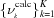corresponding to ρcalc(r) is calculated. (iii) Some measure of the similarity between the calculated and standard histograms is calculated, e.g. the coefficient of linear correlation,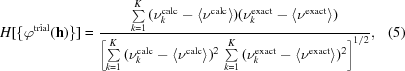where 〈〉 represents the mean value.

#### 4.2. Test phasing for RNAse Sa

The application of the selection criterion (5) is illustrated with test calculations performed with the data of the known structure of RNAse Sa (Ševcik et al., 1991). The crystals belong to space group P212121, with unit-cell dimensions a = 64.9, b = 78.32, c = 38.8 Å, and contain two molecules of 96 residues each per asymmetric unit. The 16 Å resolution set of reflections (39 independent reflections) was used for the phasing tests.

The first question is: does the highest H value correspond to the best phase set in a given population? The answer is negative. Fig. 2gives the results of the analysis of 4000 randomly generated phase sets. Every phase variant is represented on the diagram by a point whose coordinates are the phase correlation (1) with the true phases and the coefficient of linear correlation (5) between the calculated and standard histograms for the 16 Å resolution Fourier syntheses. The figure shows that in this case the best histogram agreement would result in quite bad phases and that the best phase set among the generated variants could not be recognized solely on the histogram correlation value H.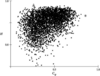Figure 2 The analysis of 4000 randomly generated 16 Å resolution phase sets (39 independent reflections each) for RNAse Sa. Every point in the diagram corresponds to one phase set and its coordinates are the map correlation coefficient Cφ and the histogram correlation coefficient H. The triangle marks the phase set resulting in the best histogram. The circle marks the phase set resulting in the map closest to the true one; this map cannot be identified by the H criterion.

An alternative analysis is shown in Fig. 3. Here, the distribution of variants in accordance with their phase quality, i.e. with their phase correlation with the true phases is given. The analysis was performed for the total random population (4000 variants) and also for the 378 variants selected by their high histogram correlation (5). The graphs show that the selected population still contains bad variants (the left tail' of the distribution), but the concentration of good variants is higher for the selected population than for the starting random population.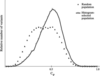Figure 3 The distribution of the variants in the start (random) and selected populations with the map correlation coefficient (in comparison with the exact 16 Å resolution map). 4000 random and 378 histogram-selected 16 Å resolution phase sets (39 independent reflections each) are analysed for RNAse Sa.

Obviously, both Fig. 2and Fig. 3can be calculated in test studies only; they are unfeasible if the true phases are unknown. Fig. 4shows the cluster tree which represents the process of step-by-step combination of the closest variants in clusters and which can be calculated without the knowledge of the true phase values. Every node corresponds to merging of two clusters and the mean variant-to-variant distance is given by the ordinate value. At the top level of the tree, the selected variants are divided into two clusters: a small cluster consisting of 52 variants and a large one consisting of 326 variants. Averaging of variants inside the large cluster with (3) has resulted in the synthesis possessing 67% correlation with the exact 16 Å resolution synthesis. Fig. 5shows the positions of the peaks in the averaged synthesis overlapped with the true positions of Cα atoms. Approximate positions of all eight molecules in the unit cell might be found in this case from the ab initio phased synthesis. Such information may be valuable in difficult cases of the search for the translation vectors in the molecular-replacement approach. At a lower cutoff, the average synthesis shows the continuous molecular region corresponding to all the molecules in the unit cell (Fig. 6). It must be noted that the molecular positions and a merged molecular region are all we can hope to extract from low-resolution syntheses of closely packed molecules.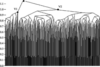Figure 4 The cluster tree for 378 histogram-selected variants for RNAse Sa (see §4.2).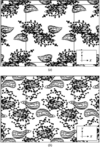Figure 5 The histogram-phased 16 Å resolution Fourier synthesis (39 independent reflections) for RNAse Sa overlapped with Cα-atom positions in (a) molecules linked to the molecule A by space-group symmetries, (b) the same for the molecule B. The projection through the unit cell along the z axis is shown. The cutoff level isolates a volume equal to 20 Å3 per residue.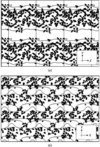Figure 6 The histogram-phased 16 Å resolution Fourier synthesis (39 independent reflections) for RNAse Sa overlapped with all atomic positions; (a) z = 0 section, (b) z = 1/4 section. The cutoff level isolates the volume equal to 200 Å3 per residue.

The averaging of variants inside the smaller cluster did not lead to any obvious molecular region (e.g. the solvent region).

The difficulties discussed above are not related to histogram-based criterion only and are present when using all known low-resolution selection criteria. Some further examples are given below.

### 5. Connectivity-based phasing

#### 5.1. Connectivity properties of low-resolution Fourier syntheses

Another example of restrictions on phase sets is topological properties of regions of high electron density, e.g. connectivity. This has been used for many years to estimate the quality of electron-density maps and was formalized as a quantitative criterion for high-resolution Fourier synthesis by Baker et al. (1993). This idea has recently been adapted for low-resolution ab initio phasing (Lunin et al., 1999, 2000).

For any function ρ(r) in the unit cell we can define a high-value region corresponding to the chosen cutoff κ as a set of all the points r in the unit cell such that ρ(r) > κ,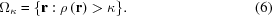We consider below the simplest properties of these regions, such as the number of components in the region Ωκ and their volume. A set of points in real space is considered as a connected component if every two points in this set may be connected by a continuous curve such that all points on the curve belong to the set.

For arbitrary chosen functions, high-value regions may have different properties. At the same time, when being selected with the use of exactly phased macromolecular Fourier syntheses, these regions reveal some common features which can be used as restrictions on phase sets. If the synthesis resolution is low and the cutoff level is high enough, it is expected that the region Ωκ consists of a small number of globs' corresponding to single molecules. When the cutoff level is lowered, these globs merge into a continuous region. At a resolution of about 2–3 Å, a high-value region may represent the trace of the polypeptide chain, while at high resolution it may consist of peaks corresponding to individual atoms. The full connectivity analysis of a function ρ(r) consists of the study of connectivity properties for the regions Ωκ corresponding to different Fourier synthesis resolutions and different cutoff levels. In this paper, we restrict consideration to the simplest case when the resolution and cutoff level are fixed.

The function ρ(r) may be calculated on different scales and with the use of different weights for individual reflections, so the use of absolute values of cutoff levels is inconvenient. For the analysis of the Fourier syntheses at low resolution, we found it convenient to fix the volume per residue in Ωκ. We say that the cutoff level κ = κ(α) corresponds to the specific volume α3 per residue) and denote the corresponding region as Ωα = Ωκ(α) if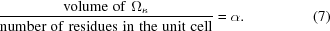If the value α is fixed, then the scale of the observed magnitudes affects the κ value, but does not change the Ωκ region.

The study of low-resolution syntheses corresponding to different macromolecules confirms the hypothesis that for a high enough cutoff level the regions Ωκ are usually compact and equal in number to the molecules in the unit cell. Naturally, the regions linked by crystallographic symmetry have exactly the same volume. If non-crystallographic symmetry is present, then the regions related by it may be slightly different in shape and volume owing to the finite resolution. Tests with known structures have shown that 25 Å3 per residue is usually a suitable volume to reveal separate globs if around 15 independent low-resolution reflections per molecule are used to calculate the Fourier synthesis. As a result, the following property of well phased low-resolution syntheses may be postulated.

#### 5.2. The selection principle

For a well phased low-resolution synthesis the number of connected components in the high-value region Ωα defined by the specific volume α = 25 Å3 per residue should be equal to the number of molecules in the unit cell. The components must have the same volume in the absence of non-crystallographic symmetry, otherwise the volume is allowed to be slightly different.

#### 5.3. The connectivity-based selection criterion

The connectivity-based selection criterion H may now be calculated for any trial phase set {φtrial(h)hS} as follows.

 (i) The Fourier synthesis ρcalc(r) is calculated with the use of the trial phases and the observed magnitudes. (ii) The cutoff level κ is determined to have the desired value of the specific volume in (7) (α = 25 Å3 per residue). (iii) The trial region Ωα is determined. (iv) The number of connected components in the region Ωα and their size is determined. (v) The trial phase set is considered as admissible and is stored if the selection principle is satisfied; the phase set is rejected otherwise.
It is worth noting that the connectivity-based selection criterion formulated above is a binary criterion, i.e. the trial phase set is either adopted or rejected unambiguously. At higher resolution the connectivity-based criterion H may be defined in a more flexible form, e.g. as the number of connected components. Variants would then be selected if H ≤ Hcrit and rejected otherwise, where Hcrit is a number specified in advance.

#### 5.4. Test phasing for γ-crystallin IIIb

The application of the connectivity-based selection criterion is illustrated by test calculations performed with data from γ-­crystallin IIIb (solved by Chirgadze et al., 1991). The crystals belong to space group P212121 with unit-cell dimensions a = 58.7, b = 69.5, c = 116.9 Å, and contain two molecules of 173 residues each per asymmetric unit. The set of reflections with d > 24 Å (28 independent reflections) was taken for phasing.

Fig. 7represents the distribution of variants according to their phase quality, i.e. their phase correlation with true phases. This analysis was performed both for a random population (100 000 variants) and for 495 variants with the desired connectivity. The graphs show the same trait as the histogram criterion in that the selected population still contains bad variants (the left `tail' of the distribution), but the concentration of relatively good variants is higher for the selected population than for the starting one. The cluster analysis shows that in this case there exists only one clear cluster, so all 495 selected variants were averaged to obtain the centroid phases and figures of merit. The corresponding Fourier synthesis had a correlation of 89% with the true 24 Å resolution synthesis. Study of this synthesis has shown (Lunin et al., 2000) that the eight highest peaks correspond to positions of the eight molecules in the unit cell, while use of a lower cutoff level shows a continuous molecular region composed of all molecules in the unit cell.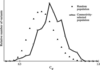Figure 7 The distribution of the variants in the start (random) and selected populations with the map correlation coefficient (in comparison with the exact 24 Å resolution map). 100 000 random and 495 connectivity-selected 24 Å resolution phase sets (28 independent reflections each) are analysed for γ-crystallin IIIb.

The high value of the map correlation coefficient could be caused by several strong reflections whose phases were chosen arbitrarily to fix the origin and enantiomorph. To estimate this effect, four such reflections were excluded from the calculation of the map correlation coefficient. The correlation value obtained for the remaining 24 reflections was 77%.

### 6. Likelihood-based phasing

#### 6.1. Likelihood-based selection criterion

Another property of the correct molecular region Ωexact is that it contains almost all the atoms of the object studied (a small fraction might be outside owing to resolution effects). At low resolution, even random atomic positions inside Ωexact may give a good approximation to the observed magnitudes. On the other hand, if the region Ω is chosen arbitrarily then the possibility of reproducing the observed magnitudes using randomly placed atoms is very low. Therefore, the probability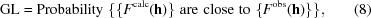where {Fcalc(h)} are calculated from the atoms randomly placed in a trial molecular region, seems to be a reasonable estimation of how correctly the region is defined. We call the value (8) the generalized likelihood, as it may be considered a generalization of the statistical likelihood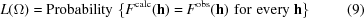corresponding to the hypothesis that the observed magnitudes are the ones calculated from the atoms randomly placed in region Ω. The search for the region with the maximum GL value (Lunin et al., 1998; Petrova et al., 1999, 2000) is similar to a search for the maximal-likelihood prior atomic coordinate distribution (Bricogne & Gilmore, 1990).

To define (8) more precisely, it is necessary to specify which sets of magnitudes are considered as close. We introduce the generalized likelihood as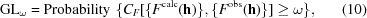where ω is the accuracy level chosen in advance and CF is the magnitude correlation coefficient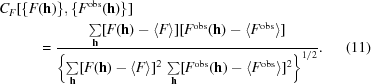A straightforward (though computationally expensive) procedure has been suggested for estimating the generalized likelihood value (Lunin et al., 1998). For a region Ω being studied, a large number of pseudo-atomic models are generated with the atomic positions inside Ω. For each model, structure factors are calculated as well as the magnitude correlation (11). The ratio of the number of models resulting in CFω to the total number of generated models gives an estimate of the probability (10).

The likelihood-based selection criterion H may now be calculated for any trial phase set {φtrial(h)}hS as follows.

 (i) The Fourier synthesis ρcalc(r) is calculated with the use of the trial phases coupled with the observed magnitudes. (ii) The trial molecular region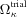is defined as the region of the highest ρcalc(r) values. (iii) The probability is estimated (by a Monte Carlo type computer simulation) to have a high correlation between the observed magnitudes and those calculated from atomic positions randomly chosen inside theregion,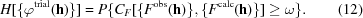As before, the idea of the phasing method is to randomly generate a number of variants and select those with a relatively high value of H[{φtrial(h)}].

#### 6.2. Test phasing for elongation factor G

Some features of the application of the likelihood-based selection criterion are illustrated with test calculations performed with data from ribosomal elongation factor G (EFG, previously solved by Ævarsson et al., 1994). The crystals belong to space group P212121, with unit-cell dimensions a = 75.9, b = 105.6, c = 115.9 Å, and contain one molecule (of 689 residues) per asymmetric unit. The 29 Å resolution set of reflections (30 independent reflections) was taken for the phasing.

To check the potential of the likelihood-based selection criterion, 696 random phase sets were generated. Fig. 8presents the analysis of the generated variants. Every variant is represented on the diagram by a point whose coordinates are the phase correlation (1) with the true phases and the generalized likelihood value (10). Again the figure shows similarities to the histogram-based criterion (Fig. 2). It follows that the search for the largest likelihood could result in quite bad phases, as the best phase set does not have the highest GL value. Nevertheless, as with histogram-based and connectivity-based criteria, the selection of variants with large H values gives a population containing a higher percentage of good variants than the random population (Petrova et al., 2000). The averaging of variants with the highest GL values resulted in a synthesis with 66% correlation with the exactly phased 29 Å resolution one.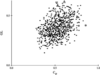Figure 8 The analysis of 696 randomly generated 29 Å resolution phase sets (30 independent reflections each) for elongation factor G. Every point in the diagram corresponds to one phase set and its coordinates are the map correlation coefficient Cφ and the generalized likelihood value GL. The triangle marks the phase set resulting in the best GL value. The circle marks the phase set resulting in the map closest to the true one; this map cannot be identified by the GL criterion.

### 7. Model-based phasing

#### 7.1. Few-atoms model method

In previous examples, every phase set in the starting population was composed of randomly and independently generated values of particular phases. The only restrictions were applied to phases of centric reflections (two allowed values). More sophisticated procedures of phase generation may be used to adopt additional information about the object studied. For example, we may randomly choose the atomic coordinates and then calculate the phases from this set of coordinates. In this case, the phases are random variables but they are no longer independent; they are linked through atomic coordinates. Another example is the calculation of the phases from a randomly rotated and translated atomic model of a homologous object. Here again the phases are random but not independent; they are linked through rotation angles and translation-vector components.

A more simple class of such models are the few-atom models (FAMs), which consist of a small number of large Gaussian spheres (Lunin et al., 1995, 1998). In this case, the coordinates of the sphere centres are the primary variables and the phases are calculated from these coordinates. In the simplest case, the model may consist of only one atom. In such a case, a regular grid of atomic centre positions can be investigated (Harris, 1994; Andersson & Hovmöller, 1996).

Another advantage of using models is that in addition to the calculated phases we have the calculated magnitudes that can be used for construction of selection criteria. For example, the magnitude correlation coefficient (11) may be used as a measure of reliability of the trial phase set. The selection criterion can now be defined as follows.

 (i) The sets of the magnitudes {Fcalc(h)} and phases {φcalc(h)} are calculated from the FAM coordinates. (ii) The value of the selection criterion is defined asThe phasing procedure can now consist of the random generation of FAMs and the selection of phase sets corresponding to FAMs with the largest values of the magnitude correlation (13).

#### 7.2. Test phasing for the AspRS–tRNAAsp complex

The test described below was performed with neutron diffraction data (Moras et al., 1983) from the cubic form of the AspRS–tRNAAsp complex (Urzhumtsev et al., 1994). The crystal belongs to space group I432, with unit-cell parameter a = 354 Å, and contains two subunits of synthetase (478 residues each) and two tRNAs (75 bases each) per asymmetric unit. The 40 Å resolution set of reflections (49 independent reflections) was used for phasing.

The simplest example of the FAM is the one-sphere model. Fig. 9shows the analysis of 1000 one-atom models with randomly generated coordinates for their centres. Every model is represented in this diagram by one point. The point coordinates are defined by the phase correlation (1) for the calculated phases {φcalc(h)} and by the magnitude correlation (11) for the calculated magnitudes {Fcalc(h)}. Again, the best value of the selection criterion corresponds to a bad variant and, vice versa, the single best variant cannot be recognized by its selection-criteria value. Thus, simply maximizing the correlation (or minimizing the R factor) between observed magnitudes and magnitudes calculated from one-sphere models is not enough to reliably determine the molecular position. The case is similar when the number of pseudo-atoms in the FAM is increased. However, we see again that selection of phase sets with high values of the selection criterion gives a population containing a higher percentage of good variants (Lunin et al., 1995, 1998).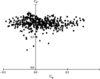Figure 9 The analysis of 1000 phase sets (AspRS–tRNAAsp complex, 40 Å resolution, 49 independent reflections) calculated with randomly generated one-atom models. Every point in the diagram corresponds to one phase set and its coordinates are the map correlation coefficient Cφ and the correlation coefficient for the calculated and observed structure-factor magnitudes CF. The triangle marks the phase set resulting in the best magnitude correlation. The circle marks the phase set resulting in the map closest to the true one; this map cannot be identified by the CF criterion.

### 8. Combination of methods: phasing for T50S

In previous sections, the suggested selection criteria were evaluated separately. An obvious step is to use the different criteria together. The methods described above were applied to the determination of the spatial structure of the ribosomal 50S particle from Thermus thermophilus. Access to the experimental X-ray diffraction data (Volkmann et al., 1990) was kindly permitted by the ribosome project leader Professor Yonath and the work was performed in collaboration with her group. The method was applied in parallel to and independently of other phasings. The initial FAM procedure produced a crystallographic image that was rather spherical and featureless and had a resolution of approximately 70 Å (Urzhumtsev et al., 1996). Dummy atoms were then generated inside the envelope and several model selection criteria were used simultaneously. Both connectivity-based and likelihood-based criteria were used at this stage to select the optimal cluster. Finally, the FAM method was applied at higher resolution using the automated procedure of the cluster selection which manipulates many more clusters and therefore includes a larger number of structure factors (Lunin et al., 1998). This last step gave an image at a resolution of roughly 40 Å.

After this solution was obtained, a model built by three-dimensional reconstruction of electron-microscopy images (Stark et al., 1995) became available. To compare these results, the phases calculated from the EM model were coupled with the observed X-ray magnitudes and the corresponding Fourier synthesis was compared with the ab initio phased synthesis. The map correlation coefficient (1) was 80% for 266 reflections in 40 Å resolution zone. Fig. 10shows views of the particle obtained with the ab initio and EM-phased syntheses.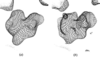Figure 10 Fourier syntheses for ribosomal particle T50S from T. thermophiluscalculated at 40 Å resolution (266 independent reflections) with X-ray experimental magnitudes and (a) ab initio determined phases, (b) phases calculated from the electron-microscopy model.

### Acknowledgements

The authors wish to express their gratitude to Yu. N. Chirgadze, E. Dodson, D. Moras and A. Yonath, who supplied the data for the test structures. The authors thank the referees and Dr J. Wilson for their valuable help in improving the manuscript. This work was supported by RFBR grants 97-04-48319 and 99-07-90461 and a CNRS Fellowship (VYL).

### ReferencesBIOLOGICALCRYSTALLOGRAPHY